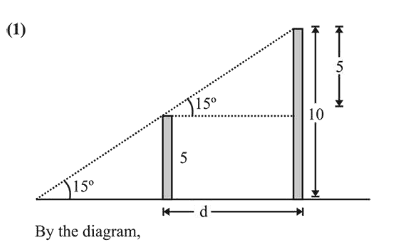# Two poles standing on a horizontal ground

Question:

Two poles standing on a horizontal ground are of heights $5 \mathrm{~m}$ and $10 \mathrm{~m}$ respectively. The line joining their tops makes an angle of $15^{\circ}$ with the ground. Then the distance (in $\mathrm{m}$ ) between the poles, is:

1. (1) $5(2+\sqrt{3})$

2. (2) $5(\sqrt{3}+1)$

3. (3) $\frac{5}{2}(2+\sqrt{3})$

4. (4) $10(\sqrt{3}-1)$

Correct Option: 1

Solution:$\tan 15^{\circ}=\frac{5}{d} \Rightarrow d=\frac{5}{\tan 15^{\circ}}=\frac{5(\sqrt{3}+1)}{\sqrt{3}-1}$

$=\frac{5(4+2 \sqrt{3})}{2}=5(2+\sqrt{3})$